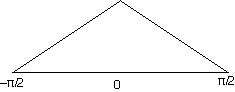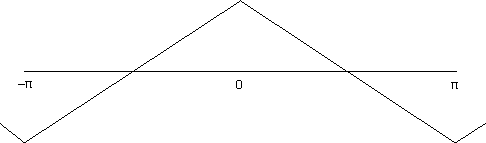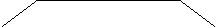Here is a solution to the vibrating string that is not widely known. I find it amusing and interesting. It can be argued that it is the most natural solution. At position x along the string and at time t the string is displaced from its rest position by y = f(x,t). The string differential equation is ∂2y/∂t2 = ∂2y/∂x2.

If the string is strung for −π/2≦x≦π/2 and to pluck we pull up the middle (x = 0) to y = 1 and then damp all movement before we release, the initial boundary conditions for t=0 are: y = 1+2x/π for −π/2≦x≦0, 1−2x/π for 0≦x≦π/2, and ∂y/∂t = 0.For the symmetry to simplify calculations we extend the problem by declaring that when t=0 and for −∞<x<∞
f(x,0) = −f(x+π,0) and therefore f(x,0) = f(x+2π,0) and f(x,t) = f(−x,t). ∂f(x,t)/∂t = 0.We will conclude that f(x,t) = f(x,−t).

The Fourier series for the initial configuration is y = ∑ ancos nx summed over positive integers. Any term in sin nx would violate f(x,t) = f(−x,t).
an = ∫ [−π, π] y cos nx dx/π.
The integral is 0 by symmetry (f(x,0) = −f(x+π,0)) when n is even.

If n is odd then ∫ [−π, π] y cos nx dx/π =
 (4/π) ∫ [−π/2, 0] y cos nx dx Each quarter of [−π,π] yields same integral = (4/π) ∫ [−π/2, 0] (1+2x/π) cos nx dx definition of y = (4/π) ∫ [0, π/2] (1+2(x−π/2)/π) cos n(x−π/2) dx (old x) = (new x)−π/2 = (4/π) ∫ [0, π/2] (2x/π) sin nx dx (−1)(n−1)/2 n is odd = (8/π2) ∫ [0, π/2] x sin nx dx (−1)(n−1)/2 factor constants = (8/π2) ∫ [0, nπ/2] (z/n) sin z dz/n (−1)(n−1)/2 x = z/n = (8/(π2n2)) ∫ [0, nπ/2] z sin z dz (−1)(n−1)/2 factor constants = (8/(π2n2))[sin z − z cos z][0, nπ/2](−1)(n−1)/2 Dwight = (8/(π2n2))[sin nπ/2 − (nπ/2) cos (nπ/2)](−1)(n−1)/2 Apply limits = (8/(π2n2))[sin nπ/2](−1)(n−1)/2 cos (nπ/2) = 0 for odd n = (8/(π2n2))[(−1)(n−1)/2](−1)(n−1)/2 sin nπ/2 = (−1)(n−1)/2 for odd n = (8/(π2n2)) Poof

f(x,0) = (8/π2)∑ cos(nx)/n2 summed over odd n. This code corroborates these tedious calculations.

(Digression: This is the first proof I learned that ∑ 1/n2 = π2/6.)

### Time Passes

We now consider the time evolution of the string. The shape of the string at time t is f(x,t) = ∑ an(t) cos nx. Each component an(t) cos nx of the Fourier series evolves according to the differential equation and it is easy to see thereby that an(t) = an(0)cos nt. We have thus f(x,t) = ∑ an(0) cos(nx)cos(nt) where
an(0) = an = (8/π2)n−2 of the previous section. Again an(0) = 0 for even n.

This expansion has a curious symmetry between t and x and indeed, f(x,t) is the height of a square pyramid on the square −π/2<x<π/2 and −π/2<t<π/2. In that square f(x,t) = min(1−2x/π, 1+2x/π, 1−2t/π, 1+2t/π). This is the 2D Fourier series for the pyramid. At t=1 the string looks thus:and some numeric corroboration.

Imagine what the topographic map of a pyramid looks like and then contemplate:This is a topographic plot of f(x,t). Each boundary between black and white is an isopleth of constant height (y). The picture is black at <x,t> if floor(25*f(x,t)) is odd. There are 25 isopleths and they describe well the square sections of a pyramid. This Java program made the picture. The picture above includes terms thru cos(59x)cos(59t). Below is the same plot with only three terms of the series (thru cos(5x)cos(5t)).Note that f(x, π/2) = 0. At t = π/2 the string is straight and all of the energy is kinetic. ∂y/∂t = −2/π for -π/1<x<π/2 but 2/π for π/2<x<3π/2.

Here is a related configuration.

There is, of course, a quicker way when you have ∂y/∂t = 0 at t = 0.
f(x,t) = (f(x−t,0) + f(x+t,0))/2.